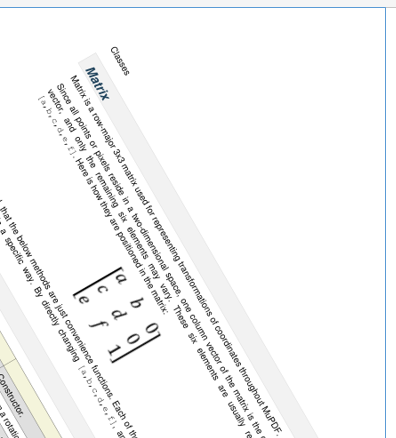# Matrix

Matrix is a row-major 3x3 matrix used by image transformations in MuPDF (which complies with the respective concepts laid down in the Adobe PDF Reference 1.7). With matrices you can manipulate the rendered image of a page in a variety of ways: (parts of) the page can be rotated, zoomed, flipped, sheared and shifted by setting some or all of just six float values.

Since all points or pixels live in a two-dimensional space, one column vector of that matrix is a constant unit vector, and only the remaining six elements are used for manipulations. These six elements are usually represented by `[a, b, c, d, e, f]`. Here is how they are positioned in the matrix: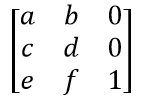Please note:

• the below methods are just convenience functions - everything they do, can also be achieved by directly manipulating the six numerical values
• all manipulations can be combined - you can construct a matrix that rotates and shears and scales and shifts, etc. in one go. If you however choose to do this, do have a look at the remarks further down or at the Adobe PDF Reference 1.7.
Method / Attribute Description
`Matrix.preRotate()` perform a rotation
`Matrix.preScale()` perform a scaling
`Matrix.preShear()` perform a shearing (skewing)
`Matrix.preTranslate()` perform a translation (shifting)
`Matrix.concat()` perform a matrix multiplication
`Matrix.invert()` calculate the inverted matrix
`Matrix.a` zoom factor X direction
`Matrix.b` shearing effect Y direction
`Matrix.c` shearing effect X direction
`Matrix.d` zoom factor Y direction
`Matrix.e` horizontal shift
`Matrix.f` vertical shift

Class API

class `Matrix`
`__init__`(self)
`__init__`(self, zoom-x, zoom-y)
`__init__`(self, shear-x, shear-y, 1)
`__init__`(self, a, b, c, d, e, f)
`__init__`(self, matrix)
`__init__`(self, degree)
`__init__`(self, list)

Overloaded constructors.

Without parameters, `Matrix(0.0, 0.0, 0.0, 0.0, 0.0, 0.0)` will be created.

`zoom-*` and `shear-*` specify zoom or shear values (float), respectively.

`matrix` specifies another `Matrix` from which a new copy will be made.

Float value `degree` specifies the creation of a rotation matrix.

Python sequence `list` (list, tuple, etc.) must contain exactly 6 values when specified. Non-numeric entries will raise an exception.

`fitz.Matrix(1, 1)`, `fitz.Matrix(0.0))` and `fitz.Matrix(fitz.Identity)` create modifyable versions of the Identity matrix, which looks like `[1, 0, 0, 1, 0, 0]`.

`preRotate`(deg)

Modify the matrix to perform a counter-clockwise rotation for positive `deg` degrees, else clockwise. The matrix elements of an identity matrix will change in the following way:

`[1, 0, 0, 1, 0, 0] -> [cos(deg), sin(deg), -sin(deg), cos(deg), 0, 0]`.

Parameters: deg (float) – The rotation angle in degrees (use conventional notation based on Pi = 180 degrees).
`preScale`(sx, sy)

Modify the matrix to scale by the zoom factors sx and sy. Has effects on attributes `a` thru `d` only: `[a, b, c, d, e, f] -> [a*sx, b*sx, c*sy, d*sy, e, f]`.

Parameters: sx (float) – Zoom factor in X direction. For the effect see description of attribute `a`. sy (float) – Zoom factor in Y direction. For the effect see description of attribute `d`.
`preShear`(sx, sy)

Modify the matrix to perform a shearing, i.e. transformation of rectangles into parallelograms (rhomboids). Has effects on attributes `a` thru `d` only: `[a, b, c, d, e, f] -> [c*sy, d*sy, a*sx, b*sx, e, f]`.

Parameters: sx (float) – Shearing effect in X direction. See attribute `c`. sy (float) – Shearing effect in Y direction. See attribute `b`.
`preTranslate`(tx, ty)

Modify the matrix to perform a shifting / translation operation along the x and / or y axis. Has effects on attributes `e` and `f` only: `[a, b, c, d, e, f] -> [a, b, c, d, tx*a + ty*c, tx*b + ty*d]`.

Parameters: tx (float) – Translation effect in X direction. See attribute `e`. ty (float) – Translation effect in Y direction. See attribute `f`.
`concat`(m1, m2)

Calculate the matrix product `m1 * m2` and store the result in the current matrix. Any of `m1` or `m2` may be the current matrix. Be aware that matrix multiplication is not commutative. So the sequence of `m1`, `m2` is important.

Parameters: m1 (Matrix) – First (left) matrix. m2 (Matrix) – Second (right) matrix.
`invert`(m)

Calculate the matrix inverse of `m` and store the result in the current matrix. Returns `1` if `m` is not invertible (“degenerate”). In this case the current matrix will not change. Returns `0` if `m` is invertible, and the current matrix is replaced with the inverted `m`.

Parameters: m (Matrix) – Matrix to be inverted. int
`a`

Scaling in X-direction (width). For example, a value of 0.5 performs a shrink of the width by a factor of 2. If a < 0, a left-right flip will (additionally) occur.

Type: float
`b`

Causes a shearing effect: each `Point(x, y)` will become `Point(x, y - b*x)`. Therefore, looking from left to right, e.g. horizontal lines will be “tilt” - downwards if b > 0, upwards otherwise (b is the tangens of the tilting angle).

Type: float
`c`

Causes a shearing effect: each `Point(x, y)` will become `Point(x - c*y, y)`. Therefore, looking upwards, vertical lines will be “tilt” - to the left if c > 0, to the right otherwise (c ist the tangens of the tilting angle).

Type: float
`d`

Scaling in Y-direction (height). For example, a value of 1.5 performs a stretch of the height by 50%. If d < 0, an up-down flip will (additionally) occur.

Type: float
`e`

Causes a horizontal shift effect: Each `Point(x, y)` will become `Point(x + e, y)`. Positive (negative) values of `e` will shift right (left).

Type: float
`f`

Causes a vertical shift effect: Each `Point(x, y)` will become `Point(x, y - f)`. Positive (negative) values of `f` will shift down (up).

Type: float

## Remarks 1

For a matrix `m`, properties `a` to `f` can also be accessed by index, e.g. `m.a == m` and `m = 1` has the same effect as `m.a = 1`. The `tuple()` and `list()` functions yield sequence objects of its components.

Language constructs like `x in m` is equal to `x in tuple(m)`.

## Remarks 2

Changes of matrix properties and execution of matrix methods can be executed consecutively. This is the same as multiplying the respective matrices.

Matrix multiplications are not commutative - changing the execution sequence in general changes the result. So it can quickly become unclear which result a transformation will yield.

To keep results foreseeable for a series of transformations, Adobe recommends the following approach (Adobe PDF Reference 1.7, page 206):

1. Shift (“translate”)
2. Rotate
3. Scale or shear (“skew”)

## Matrix Algebra

For a general background, see chapter Operator Algebra for Geometry Objects.

This makes the following operations possible:

```>>> m45p = fitz.Matrix(45)            # rotate 45 degrees clockwise
>>> m45m = fitz.Matrix(-45)           # rotate 45 degrees counterclockwise
>>> m90p = fitz.Matrix(90)            # rotate 90 degrees clockwise
>>>
>>> abs(m45p * ~m45p - fitz.Identity) # should be (close to) zero:
8.429369702178807e-08
>>>
>>> abs(m90p - m45p * m45p)           # should be (close to) zero:
8.429369702178807e-08
>>>
>>> abs(m45p * m45m - fitz.Identity)  # should be (close to) zero:
2.1073424255447017e-07
>>>
>>> abs(m45p - ~m45m)                 # should be (close to) zero:
2.384185791015625e-07
>>>
>>> m90p * m90p * m90p * m90p         # should be 360 degrees = fitz.Identity
fitz.Matrix(1.0, -0.0, 0.0, 1.0, 0.0, 0.0)
```

## Examples

Here are examples to illustrate some of the effects achievable. The following pictures start with a page of the PDF version of this help file. We show what happens when a matrix is being applied (though always full pages are created, only parts are displayed here to save space).

This is the original page image: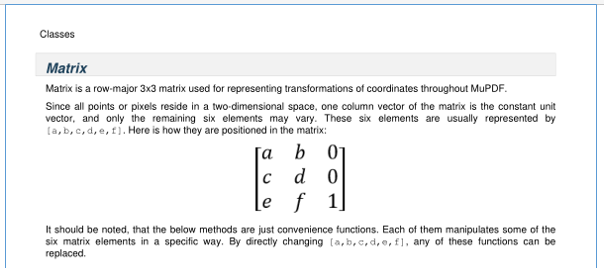## Shifting

We transform it with a matrix where `e = 100` (right shift by 100 pixels).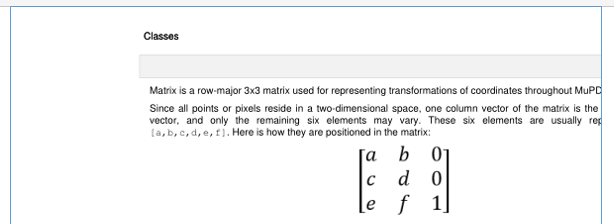Next we do a down shift by 100 pixels: `f = 100`.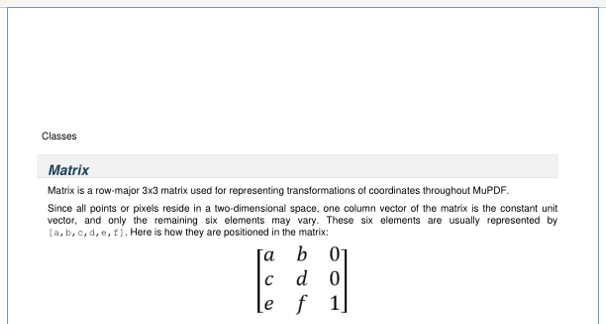## Flipping

Flip the page left-right (`a = -1`).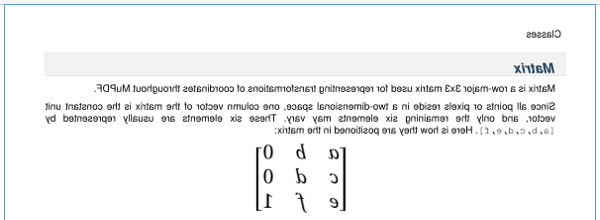Flip up-down (`d = -1`).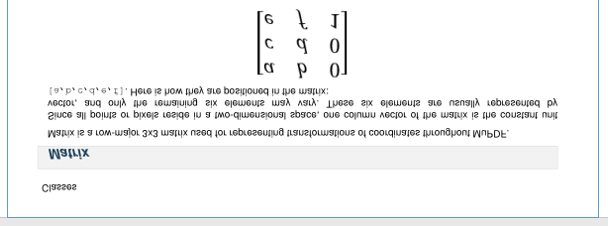## Shearing

First a shear in Y direction (`b = 0.5`).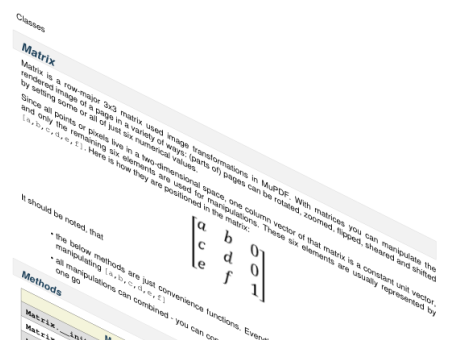Second a shear in X direction (`c = 0.5`).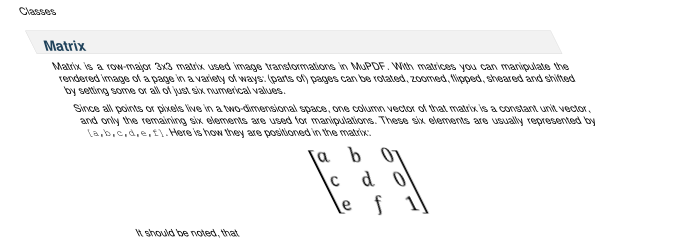## Rotating

Finally a rotation by 30 clockwise degrees (`preRotate(-30)`).